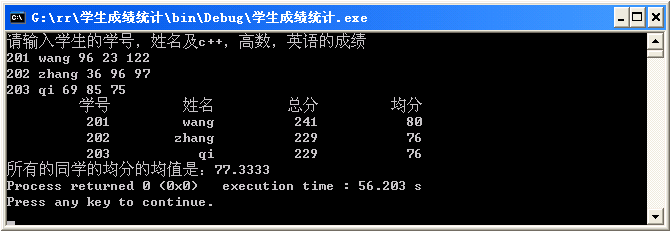• /*某班有N名同学，每个学生的信息包括学号、姓名、三门课的成绩，从键盘输入名学生的信息， 打印出N名学生三门课的平均成绩，以及最高分学生的信息（包括学号，姓名，三门课的成绩，平均分）*/ #include <stdio.h...
/*某班有N名同学，每个学生的信息包括学号、姓名、三门课的成绩，从键盘输入名学生的信息，
打印出N名学生三门课的平均成绩，以及最高分学生的信息（包括学号，姓名，三门课的成绩，平均分）*/
#include <stdio.h>
#define N 2

void Input(struct student* a);
void Output_Ave(struct student* a,double* ave);
void Output_MaxAve(struct student* a,double* ave);

struct student
{
char num;//学号
char name;//姓名
int score;//三门课成绩
};

void Input(struct student* a)
{
int i,j;
for (i=0;i<N;i++)
{
scanf ("%s %s",a[i].num,a[i].name);//输入学生的学号和姓名
for (j=0;j<3;j++)
scanf ("%d",&a[i].score[j]);//输入学生的三门课成绩
}
}

void Output_Ave(struct student* a,double* ave)
{
int i,j;
for (i=0;i<N;i++)
{
for (j=0;j<3;j++)
ave[i]+=a[i].score[j];
ave[i]/=3.0;//计算平均成绩
}
printf ("各学生三门课的平均成绩为:");//输出平均成绩
for (i=0;i<N;i++)
printf ("%lf ",ave[i]);
printf ("\n");
}

void Output_MaxAve(struct student* a,double* ave)
{
int i,index;//index表示最高分的学生索引
double max;
max=ave;
for (i=1;i<N;i++)
if (ave[i]>max)
{
max=ave[i];
index=i;
}
printf ("最高分的学生数据为:");
printf ("%s %s ",a[index].num,a[index].name);
for (i=0;i<3;i++)
printf ("%d ",a[index].score[i]);
printf ("%lf\n",max);
}

void main(void)
{
struct student a[N];
double ave[N]={0};//每名学生三门课的平均成绩
Input(a);//从键盘输入N名学生的信息
Output_Ave(a,ave);//打印出N名学生三门课平均成绩
Output_MaxAve(a,ave);//打印出最高分的学生数据
}




展开全文• 从键盘输入同学的英文（小写输入，假设学生的英文只包含3个字母。如: tom），编写程序在屏幕上输出该同学的英文，且首字母大写（如: Tom）。同时输出组成该英文的所有英文字符在26个英文字母中的序号。 ...
从键盘输入某同学的英文名（小写输入，假设学生的英文名只包含3个字母。如: tom），编写程序在屏幕上输出该同学的英文名，且首字母大写（如: Tom）。同时输出组成该英文名的所有英文字符在26个英文字母中的序号。
以下为程序的运行结果示例：
tom
Tom
t:20
o:15
m:13
输入格式： “%c%c%c”
输出格式：
首字母大写的英文姓名的输出格式："%c%c%c\n"
姓名中每个字母在26个英文字母中的序号的输出格式："%c:%d\n"
#include <stdio.h>
int main()
{char A,a,b,c;
scanf("%c%c%c",&a,&b,&c);
A=a-32;
printf("%c%c%c\n%c:%d\n%c:%d\n%c:%d\n",A,b,c,a,a-96,b,b-96,c,c-96);
return 0;}



展开全文c#
/*
*文件名称：
*作者：王争取
*完成日期：2014.12.21
*版 本 号：v1.0
*问题描述：求出每名同学的总分和均分，并存储在结构体数组中
*输入描述：从键盘上输入N名学生的信息（N定义为常变量）
*程序输出：输出每位同学的信息学号、姓名、总分和均分。
*/
#include <iostream>
#include <iomanip>
using namespace std;
struct Student
{
char num;
char name;
int cpp;
int math;
int english;
int score;
double average;
};
const int N=3;
int main( )
{
int i, j, k;
Student stu[N];//定义结构体数组
cout<<"请输入学生的学号，姓名及c++，高数，英语的成绩"<<endl;
for( i=0; i<N; i++)
{
cin>>stu[i].num>>stu[i].name>>stu[i].cpp>>stu[i].math>>stu[i].english;
stu[i].score=stu[i].cpp+stu[i].math+stu[i].english;
stu[i].average=stu[i].score/3;
cout<<endl;
}
cout<<setw(13)<<"学号"<<setw(13)<<"姓名"<<setw(13)<<"总分"<<setw(13)<<"均分"<<endl;
for(j=0; j<N; j++)
cout<<setw(13)<<stu[j].num<<setw(13)<<stu[j].name<<setw(13)<<stu[j].score<<setw(13)<<stu[j].average<<endl;
double average1,s=0;
for(k=0;k<N;k++)
s+=stu[k].average;
average1=s/N;
cout<<"所有的同学的均分的均值是："<<average1;
return 0;
}展开全文基础编程
• 4.2 定义二维数组储存m学生n门功课的成绩，m和n的值及成绩都从键盘输入，然后计算每个同学的平均成绩。要求捕获以下几种异常： （1）类型不匹配异常，如输入的是字符串等。 （2）输入的成绩不在正常范围内，如成绩...
第4章
课后习题练习
4.1 输入一个整数表示星期几，输出对应的英文单词。当输入的数据类型不匹配时（如输入的是字符串）会抛出异常，要求捕获该异常，显示提示信息“输入的数据类型不匹配”
4.2 定义二维数组储存m名学生n门功课的成绩，m和n的值及成绩都从键盘输入，然后计算每个同学的平均成绩。要求捕获以下几种异常：
（1）类型不匹配异常，如输入的是字符串等。
（2）输入的成绩不在正常范围内，如成绩>100或成绩<0
（3）计算平均成绩时，要考虑被0除的异常
3.face()方法的功能时求n!，阶乘是byte类型。当n!的值超出byte数据类型的范围时，抛出异常。在主方法中输入n的值，调用face()方法求n!的值。哟啊求风别用以下三种方式进行异常处理：
（1）fact()方法捕获异常
（2）fact()方法声明异常，主方法捕获异常
（3）fact()方法和主方法都声明异常
4.4 自定义异常类TriangleException表示三条边无法构成三角形的异常。在主方法中，输入三个整数，如果能构成三角形则求其周长和面积；如果无法构成三角形则抛出TriangleException异常，要求捕获这个异常，输出信息“输入的三条边不能构成三角形”
public class Week {

public static void main(String[] args) {
try {
int n;
String day = null;
Scanner s = new Scanner(System.in);
System.out.println("请输入想要查询的星期数：");
n = s.nextInt();
if (n == 1)
day = "Monday";
else if (n == 2)
day = "Tuesday";
else if (n == 3)
day = "Wednesday";
else if (n == 4)
day = "Thusday";
else if (n == 5)
day = "Friday";
else if (n == 6)
day = "Saturday";
else if (n == 7)
day = "Sunday";
else
throw new OutOfRangeException();
System.out.print(day);
} catch (InputMismatchException e) {
System.out.print("输入类型错误！");
} catch (OutOfRangeException e) {
System.out.print("输入数字错误！");
}
}
}
class OutOfRangeException extends Exception{
OutOfRangeException(){
super("输入类型错误");
}
}

4.2
public class Grade {

public static void main(String[] args) {
int m,n;
Double[][] g;
Scanner s = new Scanner(System.in);
System.out.print("请输入学生个数和考试科目数：");
try {
m = s.nextInt();
n = s.nextInt();
if(n == 0)
throw new ZeroException();
else {
g = new Double[m][];
for(int i = 0;i<m;i++) {
g[i] = new Double[n + 1];
g[i][n] = 0.00;
for(int j = 0;j<n;j++) {
g[i][j] = s.nextDouble();
if(g[i][j] <= 100 && g[i][j] >= 0)
g[i][n] = g[i][n] + g[i][j];
else
throw new OutOfRangeException();
}
}
for(int i = 0;i<m;i++) {
g[i][n] = g[i][n] / n;
System.out.print("\n" + "第" + (i + 1) + "个同学的平均成绩为：");
System.out.printf("%.2f",g[i][n]);
}
}

}catch(InputMismatchException e) {
System.out.print("输入类型有误！");
}catch(OutOfRangeException e) {
System.out.print("输入成绩范围有误！");
}catch(ZeroException e) {
System.out.print("无法计算平均成绩！");
}
}
}
class OutOfRangeException extends Exception{
OutOfRangeException(){
super("输入类型");
}
}
class ZeroException extends Exception{
ZeroException(){
super("0除");
}
}

4.3
public class Face {

int n;
byte sum = 1;
//	方一：
void face(int n){
try {
this.n = n;
if(n > 0) {
for(int i = 1;i <= n;i++)
sum = (byte) (sum * i);
if(sum > 0)
System.out.print("阶乘值为：" + sum);
else
throw new OutOfRangeException();
}else
throw new MismatchException();
}catch(OutOfRangeException e) {
System.out.print("超出byte范围！");
}catch(MismatchException e) {
System.out.print("输入数值有误无法计算！");
}
}
//	方二，方三的face()方法一样：
//	void face(int n) throws OutOfRangeException,MismatchException{
//			this.n = n;
//			if(n > 0) {
//				for(int i = 1;i <= n;i++)
//					sum = (byte) (sum * i);
//				if(sum > 0)
//				System.out.print("阶乘值为：" + sum);
//				else
//					throw new OutOfRangeException();
//			}else
//				throw new MismatchException();
//	}
public static void main(String[] args) {
int n;
Scanner s = new Scanner(System.in);
System.out.print("请输入n的值：");
n = s.nextInt();
Face f = new Face();
f.face(n);

}
//	方二：
//	public static void main(String[] args) {
//		int n;
//		Scanner s = new Scanner(System.in);
//		System.out.print("请输入n的值：");
//		n = s.nextInt();
//		Face f = new Face();
//		try {
//			f.face(n);
//		} catch (OutOfRangeException e) {
//			e.printStackTrace();
//		} catch (MismatchException e) {
//			e.printStackTrace();
//		}
//	}
//	方三：
//	public static void main(String[] args) throws OutOfRangeException, MismatchException {
//		int n;
//		Scanner s = new Scanner(System.in);
//		System.out.print("请输入n的值：");
//		n = s.nextInt();
//		Face f = new Face();
//		f.face(n);
//	}

}
class OutOfRangeException extends Exception{
OutOfRangeException(){
}
}
class MismatchException extends Exception{
MismatchException(){
}
}

4.4
public class Triangle {

public static void main(String[] args) {
int a,b,c;
double l,s;
Scanner sc = new Scanner(System.in);
System.out.println("请输入三边的长度：");
a = sc.nextInt();
b = sc.nextInt();
c = sc.nextInt();
try {
if((a + b)> c && (a + c)>b && (b + c)>a) {
l = a + b + c;
s = Math.sqrt((l/2 * (l/2 - a) * (l/2 - b) * (l/2 - c)));
System.out.print("该三角形的周长为：" + l + "\n面积为：" );
System.out.printf("%.2f",s);
}
else
throw new TriangleException();
}catch(TriangleException e) {
System.out.print("输入的三条边不能构成三角形");
}
}
}
class TriangleException extends Exception{
TriangleException(){
super("无法构成三角形");
}
}



展开全文java
• 从键盘输入N名同学的成绩； 求出每名同学的总成绩和平均分； 输出每名同学的信息，包括学号，姓名，总分，平均分； 按照c语言成绩排序输出 #include <stdio.h> #include <stdlib.h> #define N 2//...
• 返回：贺老师课程教学链接【项目1-学生成绩统计】 每位同学的信息学号、姓名、C、...（1）从键盘输入N名学生的信息（N定义为常变量）； （2）求出每名同学的总分和均分，并存储在结构体数组中（可以读入过程中c语言
• 返回：贺老师课程教学链接【项目1-学生成绩统计】 每位同学的信息学号、姓名、C、...（1）从键盘输入N名学生的信息（N定义为常变量）； （2）求出每名同学的总分和均分，并存储在结构体数组中（可以读入过程中
• 项目要求： 每位同学的信息学号、姓名、C++、高数、英语...（1）从键盘输入N名学生的信息（N定义为常变量）； （2）求出每名同学的总分和均分，并存储在结构体数组中（可以读入过程中“顺便”计算）； （3）输出每位
• /* *作者：刘慧艳 *完成日期：2014.03.07 *版本号：V1.0 *问题描述：每位同学的信息学号、姓名、C++、高数、英语成绩，定义一个学生成绩的结构体...　（1）从键盘输入N名学生的信息（N定义为常变量）； 　（2
• 从键盘输入同学的英文（小写输入，假设学生的英文只包含3个字母。如: tom），编写程序在屏幕上输出该同学的英文，且首字母大写（如: Tom）。同时输出组成该英文的所有英文字符在26个英文字母中的序号。 ...c
• 每位同学的信息学号、姓名、C++、高数、...（1）从键盘输入N名学生的信息（N定义为常变量）； （2）求出每名同学的总分和均分，并存储在结构体数组中（可以读入过程中“顺便”计算）； （3）输出每位同学的信息学号
• 每位同学的信息学号、姓名、C++、高数、...（1）从键盘输入N名学生的信息（N定义为常变量）； （2）求出每名同学的总分和均分，并存储在结构体数组中（可以读入过程中“顺便”计算）； （3）输出每位同学的信息学号c++ cpp
• 每位同学的信息学号、姓名、C++、高数、...（1）从键盘输入N名学生的信息（N定义为常变量）； （2）求出每名同学的总分和均分，并存储在结构体数组中（可以读入过程中“顺便”计算）； （3）输出每位同学的信息学号
• 问题及代码：/* ... *ALL right reserved *文件名：学生成绩统计 *作者；童宇 ...*完成日期：2014年12月21日 ...*输入描述：从键盘输入N名学生的信息（N定义为常变量,N==3） *程序输出：输出每位同学的信息学号、姓名、结构体 c++ 排序
• 问题及代码：/* ...*ALL right reserved *文件名：学生成绩统计 *作者；童宇 ...*输入描述：从键盘输入N名学生的信息（N定义为常变量,N==3） *程序输出：输出每位同学的信息学号、姓名、总分和均分。 */结构体 c++
• 【题目1-学生成绩统计】  每位同学的信息学号、姓名、C++、高数、英语成绩，定义一个...　（1）从键盘输入N名学生的信息（N定义为常变量）； 　（2）求出每名同学的总分和均分，并存储在结构体数组中（可以
• 问题描述： 每位同学的信息学号、姓名、C++、高数、英语成绩。...（1）从键盘输入 N 名学生的信息（N 定义为常变量）； （2）求出每名同学的总分和均分，并存储在结构体数组中（可以读入过程中“顺便”计算）；c++ namespace
• 问题描述： 每位同学的信息学号、姓名、C++、高数、英语成绩。...（1）从键盘输入 N 名学生的信息（N 定义为常变量）； （2）求出每名同学的总分和均分，并存储在结构体数组中（可以读入过程中“顺便”计算）；c++ namespace
• 问题描述： 每位同学的信息学号、姓名、C++、高数、英语成绩。...（1）从键盘输入 N 名学生的信息（N 定义为常变量）； （2）求出每名同学的总分和均分，并存储在结构体数组中（可以读入过程中“顺便”计算）；c++ namespace
• printf("\n以下是%d个同学的信息\n", n); printf("学号 姓名 性别 年龄 出生年月 电话 地址 E-Mail\n"); for (p = stu; p<stu + n; p++) printf("%d %s %c %d %d-%d %d %s %s\n", p->num, p->name, p->sex,...c c++
• /* *作者：刘慧艳 *完成日期：2014.03.07 *版本号：V1.0 *问题描述：每位同学的信息学号、姓名、C++、高数、英语成绩，定义一个学生成绩的结构体...　（1）从键盘输入N名学生的信息（N定义为常变量）； 　（2